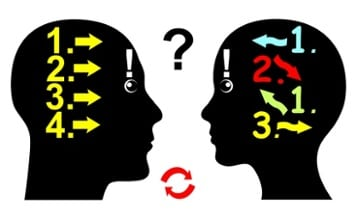# 4 Typical Student Misconceptions Math Course Writers Must ConsiderBeing familiar with common student misconceptions not only opens a window into student thinking, but also encourages math course writers to develop effective strategies to help students avoid incorrect generalizations or computational errors. Here are four common misconceptions that math course writers must consider when deciding on the best approach for a new lesson or assessment item:

1)      Math is a set of rules and procedures

This popular misconception is not without cause. Content writers and instructors alike provide steps and rules in hopes of making particular math concepts easier to grasp. In turn, students often make the error of assuming that, because a particular procedure works in one scenario, it should always work. This yearn to overgeneralize leads to students memorizing algorithms and relationships rather than developing their mathematical reasoning skills. Yes, students should be able to look for patterns and make generalizations, but they shouldn’t limit these connections to procedural knowledge. Consider how you can provide a conceptual framework for math content that exposes students to mathematical reasoning rather than perpetuate the misconception that an algorithm is a silver bullet.

2)      What it means to be exact vs. approximateAs one of the Standards for Mathematical Practice, learning what it means to “attend to precision” can be tricky for students regardless of age or content level. This may be because they lack a clear understanding of what it means for an answer to be “exact.” For example, many students believe that 3.14 is a more exact representation of the number π because it is a tangible value. While you are creating content, think about how you can help students understand how to express solutions that are accurate and appropriately precise for the context at hand. Perhaps highlighting the use of the ≈ symbol instead of the = sign when rounding a final answer can help students draw a stronger connection.

3)      The final answer is what matters, not the mathematical process behind it

Teachers have been fighting for years for students to show their work. While students may try to argue that arriving at a correct solution means they’ve mastered a concept, the new standards require students to demonstrate understanding through multiple solution methods and justification. Consider the stimulus “Leslie earns \$350 per week. She receives a 15% raise.” The question traditionally associated with this prompt would be “How much is her new weekly pay rate?” but on a next-generation assessment, students are instead asked “How can Leslie calculate her new weekly pay rate?” Therefore, it is important that math course writers design lessons and items that assess how students arrive at a solution—not just whether or not a student gets the correct answer.

4)      Math concepts are discreteOftentimes, mathematical content is introduced in such a way that students believe they are learning something new every single lesson. This is not just overwhelming for students, it’s detrimental to their critical thinking skills. It is important for math course writers to take time to make connections to previous math knowledge or let students draw their own connections. How can you relate a new concept that seems completely foreign to students, like a logarithm, to something they already know? Perhaps you connect the inverse relationship between exponentials and logarithms to that of quadratics and square roots—one undoes the other. Understanding how the content you are developing is vertically aligned to previous and future concepts also provides greater opportunity for you to debunk the myth that math is a random assortment of rules and procedures.

Analyzing these student misconceptions before developing content doesn’t just help math course writers address pedagogical shortcomings; it also provides a rich opportunity to better your own instructional practice.